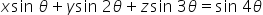Maths-
General
Easy

###Hint:

In this question, we have to find the roots of the given equation. we will assume a, b, c to be the roots. Later, we will solve the equation using some trigonometric identity and find the required roots.

## Book A Free Demo+91